Next: 6.5 Complex Functions Up: 6. The Complex Numbers Previous: 6.3 Roots of Complex   Index

# 6.4 Square Roots

Let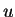be a direction in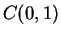, with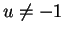. Then we know that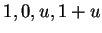are the vertices of a parallelogram.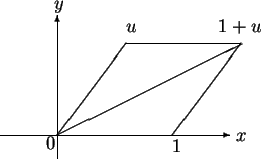Since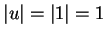, all four sides of the parallelogram are equal, and thus the parallelogram is a rhombus. Since the diagonals of a rhombus bisect its angles, the segment from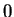to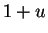bisects angle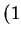--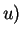. Hence I expect that the direction of(i.e.,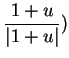is a square root of. I can prove that this is the case without using any geometry.

6.23   Theorem. Letbe a direction in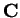with. Thenis a square root of.

Proof: I just need to square. Well,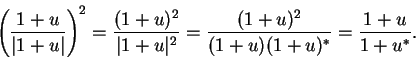Now sinceis a direction, we know that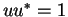, and hence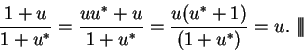6.24   Corollary. Every complex number has a square root.

Proof: Let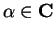. If, then clearly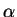has a square root. If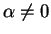, let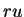be the polar decomposition for. If, then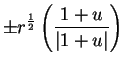are square roots of. If, thenare square roots of.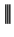6.25   Example. We will find the square roots of. Let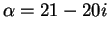. Then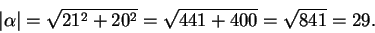Hence the polar decomposition foris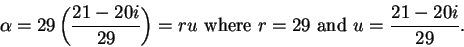The square roots ofare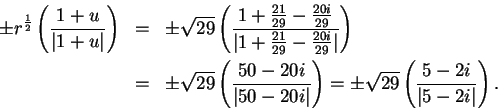Now, so the square roots ofare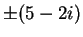.

6.26   Exercise. Find the square roots of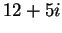. Write your answers in the form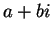, where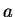and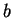are real. A

Let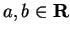. There is a formula for the square root ofthat allows you to say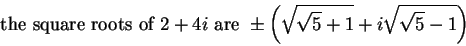(6.27)

and(6.28)

6.29   Exercise. Verify that assertions (6.27) and (6.28) are correct.

6.30   Entertainment. Find the square root formula, and prove that it is correct. (There are at least three ways to do this. Method c) is probably the easiest.)
a)
Suppose the square root is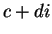, and equate the real and imaginary parts of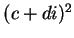and. Then solve for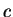andand show that your solution works.
b)
Letbe the polar decomposition of. You know how to find a square root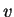for, andwill be a square root of. Write this in the form.
c)
On the basis of (6.27) and (6.28), guess the formula, and show that it works.Next: 6.5 Complex Functions Up: 6. The Complex Numbers Previous: 6.3 Roots of Complex   Index